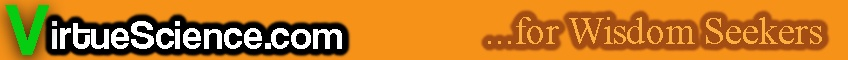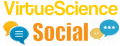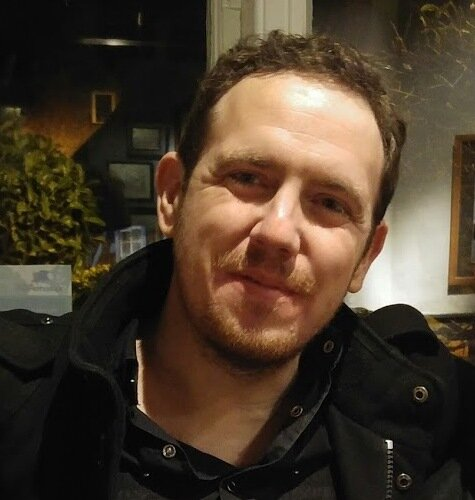Database of Number Correlations The purpose of this section is to discover unexpected connections between various material and occult phenomena. A great resource for mathematicians. Notable Events for over 2000 years of history: Discover unusual synchronicities in World History. 2500 plus pages.
Great Mathematician Ramanujan was helped by?
Which Number is both a Square Number and a Square Pyramidal Number?
I am an odd number. Take away one letter and I become even. What number am I?
Infinity plus infinity equals....?
Has the Use of Calculators Weakened Human Minds?
How Many Mathematical Axioms are There?
What is the Impact of Artificial Intelligence on Mathematics?
What is the Most Interesting Unsolved Maths Question?
Do you have a Favourite Number?
The Database of Number Correlations
The Main Number Sections:
0 - 500 | 501 - 1000 | 1001 - 1500 | 1501 - 2000 | 2001 - 2500
Number Articles | Higher Numbers | Random Number
36 37 38

# The Number 37: Properties and Meanings

37 is a Prime Number.

37 is a Centered Hexagonal Number.

37 is a Centered 12-gonal Number.

37 is the least number of 5th powers needed to represent every possible integer.

37 is the maximum number of 5th powers needed to sum to any number.

37 can be Partitioned 21637 ways.

37 can be Partitioned 19 times with each term no larger than 2.

37 can be Partitioned 133 times with each term no larger than 3.

37 can be Partitioned 511 times with each term no larger than 4.

37 can be Partitioned 1342 times with each term no larger than 5.

37 is the maximal number of regions into which 8 lines divide a plane.

The Chemical Element Rubidium has an atomic number of 37.

The Human hand is moved by 37 skeletal muscles.

In the year 37 AD Tiberius died and the mad Caligula succeeded him.

36 37 38
The Main Number Sections:
0 - 500 | 501 - 1000 | 1001 - 1500 | 1501 - 2000 | 2001 - 2500
Number Articles | Higher Numbers | Random NumberCheck out the latest Number / Mathematics Forum Topics:Hi, I am James Barton an independent truth seeker.
VirtueScience is a unique philosophy and set of tools which I have developed over many years. It combines deep new insights with ancient wisdom.
Main Article Sections